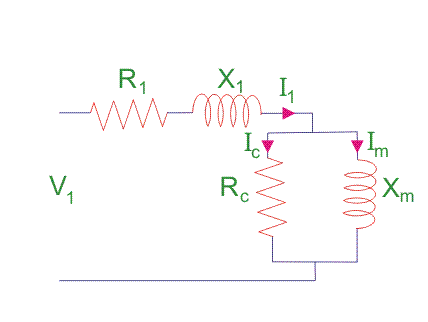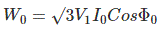# What is No Load Test of an Induction Motor?

Posted on

The no-load test of induction motor is performed to find out the constant losses occurring in the motor. What is the purpose to perform the no load test of an induction motor? Purpose of no load test of induction motor is “When the motor is running without any load or no feeder of motor energy is installed is a “No load” condition of motor”.

The main purpose to perform no load testing of an induction motor are as follows:

1. To determine the magnitude of various losses occurring in the motor.
2. For power factor measurement, two watt meters of single phase are employed to perform the readings.

# What is No Load Test of an Induction Motor?

The efficiency of large motors can be determined by directly loading them and by measuring their input and output powers. For larger motors it may be difficult to arrange loads for them. Moreover power loss will be large with direct loading tests.

Thus no load and blocked rotor tests are performed on the motors. As the name suggest no load test is performed when rotor rotates with synchronous speed and there is no load torque. This test is similar to the open circuit test on transformer.

Actually, to achieve synchronous speed in an induction motor is impossible. The speed is assumed to be synchronized. The synchronous speed can be achieved by taking slip = 0 which creates infinite impedance in the rotor branch.

This test gives the information regarding no-load losses such as core loss, friction loss and windage loss. Rotor copper loss at no load is very less that its value is negligible. Small current is required to produce adequate torque. This test is also well-known as running light test. This test is used to evaluate the resistance and impedance of the magnetizing path of induction motor.

## Theory of No Load Test of Induction Motor

The impedance of magnetizing path of induction motor is large enough to obstruct flow of current. Therefore, small current is applied to the machine due to which there is a fall in the stator-impedance value and rated voltage is applied across the magnetizing branch.

But the drop in stator-impedance value and power dissipated due to stator resistance are very small in comparison to applied voltage. Therefore, there values are neglected and it is assumed that total power drawn is converted into core loss.

The air gap in magnetizing branch in an induction motor slowly increases the exciting current and the no load stator I2R loss can be recognized.

One should keep in mind that current should not exceed its rated value otherwise rotor accelerates beyond its limit.

The test is performed at poly-phase voltages and rated frequency applied to the stator terminals. When motor runs for some times and bearings get lubricated fully, at that time readings of applied voltage, input current and input power are taken. To calculate the rotational loss, subtract the stator I2R losses from the input power.## Calculation of No Load Test of Induction Motor

Let the total input power supplied to induction motor be W0 watts.Where,

• V1 = line voltage
• I0 = No load input current

Rotational loss = W0 – S1

Where,

• S1 = stator winding loss = Nph I2 R1
• Nph = Number phase

The various losses like windage loss, core loss, and rotational loss are fixed losses which can be calculated by:

Stator winding loss = 3Io2R1

Where,

• I0 = No load input current
• R1 = Resistance of the motor

Core loss = 3GoV2